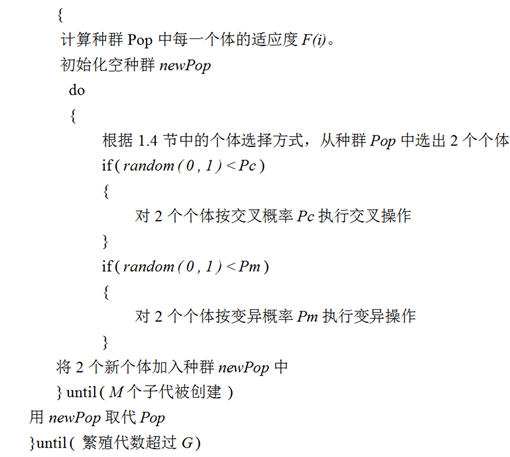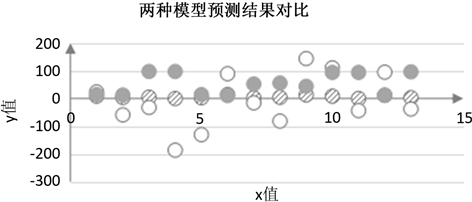# 一种基于遗传算法的回归模型寻优方法A Regression Model Optimization Method Based on Genetic Algorithm

DOI: 10.12677/SEA.2021.101002, PDF, HTML, XML, 下载: 53  浏览: 137  国家自然科学基金支持

Abstract: Regression analysis is an important tool for data analysis and modeling, mainly used for data pre-diction and fitting. Regression analysis usually requires manual intervention of a given reference model followed by parametric regression. However, in most cases, it was difficult for the user to given a reference model or given the model a large error. It proposed a method based on genetic algorithm to obtain regression model. It mainly used the idea of genetic evolution to first randomly generated an initial model populations; then iteratively selected, crossed, and mutated operations, perform global optimization dynamically in the solution space to find a better model; in order to determine the parameters of the model, the gradient descent method is used to estimate the parameters of the model. Finally, the model obtained in this paper is compared with the model obtained by least squares regression analysis. The results show that the error of the former is sig-nificantly reduced from the previous one, from 14.24% to 9.59%.

1. 引言

2. 方法设计

2.1. 问题定义

2) 可供选择的基本单目运算符：“x”，“x2”，“ $\sqrt{x}$ ”，双目运算符号：“+”、“−”。

3) 双目运算符的个数N (决定回归方程的长短，N越大，回归方程越长)。

2.2. 个体编码

2.2.1. 编码长度

$N=\left\{\begin{array}{l}2\text{ }\text{ }\text{ }\text{ }\text{ }n=0\\ 2\ast N+2\text{ }\text{ }n>0\end{array}$ (1)

2.2.2. 编码规则

2.3. 交叉和变异因子

2.4. 个体的选择方式

$p\left(i\right)=\frac{F\left(i\right)}{TotalF}$ (2)

2.5. 建立回归模型的基本步骤

Step1：根据遗传算法参数初始化的历史经验，对种群规模(M)、交叉发生的概率(Pc)、变异发生的概率(Pm)、终止进化的代数(G)进行初始化。根据1.2节编码规则，随机产生第一代初始种群Pop

Step2：doStep3：根据Step2产生的模型，用梯度下降法进行参数回归。初始化迭代步数为S，模型参数为 ${\theta }_{\text{1}},{\theta }_{\text{2}},\cdots$

Step4：迭代更新这些参数使目标函数J(θ)不断变小，直到迭代次数到达S停止迭代，J(θ)的计算如公式(3)所示：

$J\left(\theta \right)=\frac{1}{2}\underset{i=1}{\overset{m}{\sum }}{\left(h\left({x}^{i}\right)-{y}^{i}\right)}^{2}$ (3)

3. 实验与结果分析

3.1. 实验过程

3.1.1. 基于遗传算法的回归模型寻优方法Table 2. Relationship between reaction rate and reactant content

3.1.2. 最小二乘法回归分析方法

“统计产品与服务解决方案(spss)  ”软件可以对数据进行回归分析，使用该软件对化学动力学反应数据进行最小二乘法回归分析得到的结果如图1所示。t值是单样本检验，展现了该自变量对因变量是否有显著性影响，最后一列的t值所对应的Sig值，如果小于0.05，代表该自变量对结果的影响程度越高，表中系数一列表达了该自变量在回归方程中的系数，如果系数为正，该自变量与因变量则为正比例的关系；如果系数为负数，那么自变量与因变量就为反比例的关系。Figure 1. Coefficient

3.2. 两种模型预测应用Table 3. Comparison of prediction results of two modelsFigure 2. Comparison of prediction results of two models

$RMSE=\sqrt{MSE}=\sqrt{\frac{1}{n}\underset{i=1}{\overset{n}{\sum }}{w}_{i}{\left({y}_{i}-{y}_{i}^{^}\right)}^{2}}$ (4)

4. 结语

  张钰珩. 浅析回归分析在经济金融领域的运用[J]. 商场现代化, 2018(1): 147-148.  王曼. 医学论文中常用回归分析方法的审核要点及对策[J]. 编辑学报, 2018, 30(5): 475-477.  孔德兵, 尚可政, 王式功, 等. 基于逐步回归分析的西北地区东部雷暴概率预报方法研究[J]. 干旱气象, 2016, 34(1): 181-187.  孙克, 徐中民. 基于地理加权回归的中国灰水足迹人文驱动因素分析[J]. 地理研究, 2016, 35(1): 37-48.  施龙青. 基于多元回归分析法预测断层防隔水煤柱宽度[J]. 煤炭科学技术, 2013, 41(6): 108-110.  卢骏, 戴吾蛟, 章浙涛. 大坝变形变系数回归建模[J]. 武汉大学学报(信息科学版), 2015, 40(1): 139-142.  Nijhout, F. (1997) An Introduction to Genetic Algorith-MS. Complexity, 2, 39-40.https://doi.org/10.1002/(SICI)1099-0526(199705/06)2:5<39::AID-CPLX8>3.0.CO;2-L  蔡良伟, 李霞. 遗传算法交叉操作的改进[J]. 系统工程与电子技术, 2006, 28(6): 925-928.  周祥, 何小荣, 陈丙珍. 基于最优变异因子的遗传算法在ANN训练中的应用[J]. 清华大学学报(自然科学版), 2002(5): 619-621.  马洁莹. 基于轮盘赌策略的混沌萤火虫算法研究[D]: [硕士学位论文]. 西安: 西安电子科技大学, 2018.  黄文霞, 李民. 基于SPSS数据分析的影响旅游地区发展的主要因素分析[J]. 软件, 2019, 40(1): 152-157.